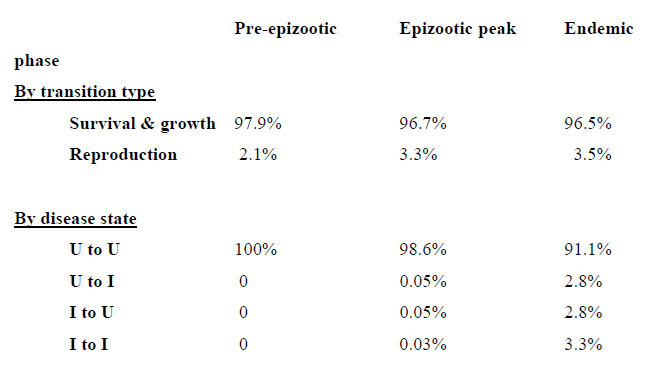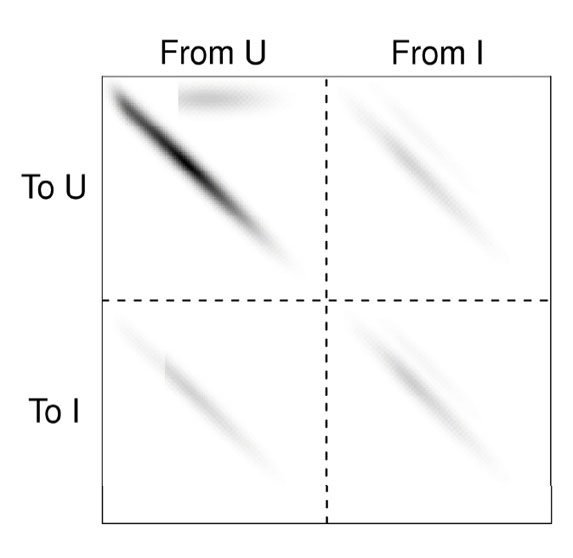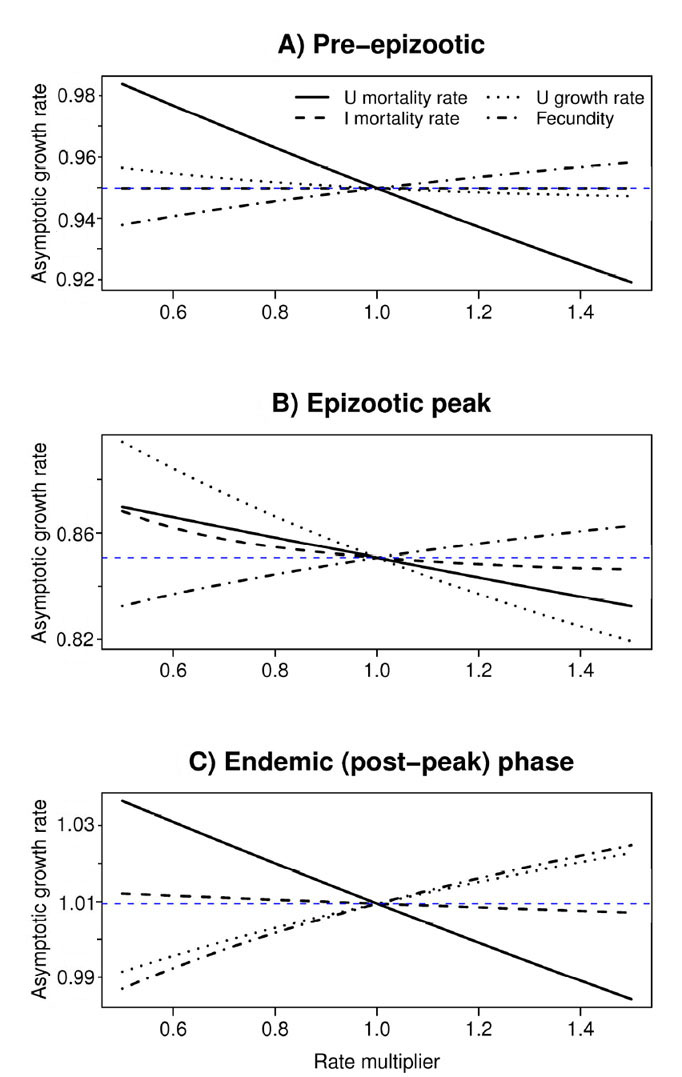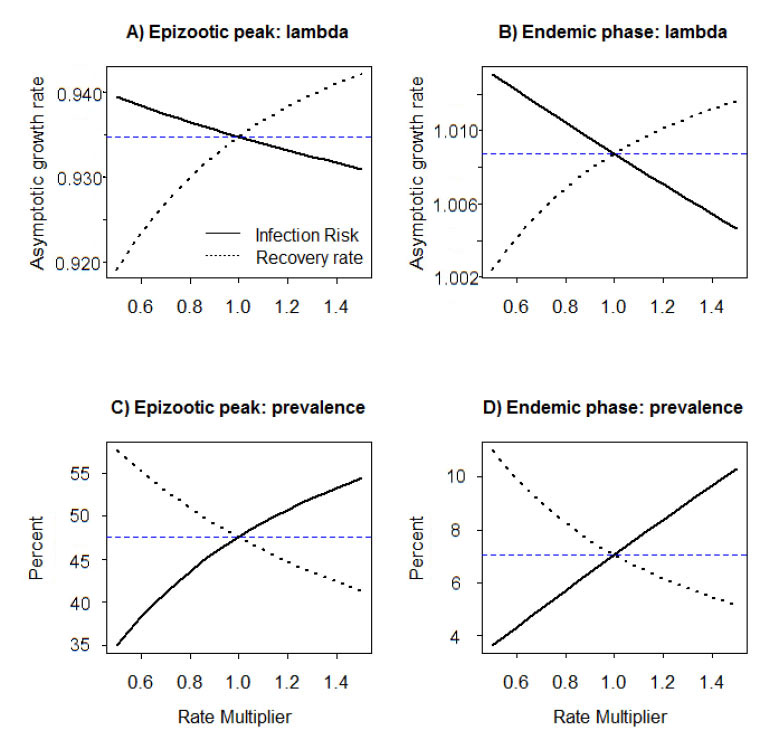### John F. Bruno, Stephen P. Ellner, Ivana Vu, Kiho Kim, and C. Drew Harvell. 2011. Impacts of aspergillosis on sea fan coral demography: modeling a moving target. Ecological Monographs 81:123–139.

Appendix E. Elasticity and perturbation analyses.

 TABLE E1. Elasticity analyses of the population model, with parameters corresponding to different stages in the epizootic.Note: Tabulated values are percentages of the total elasticity of the dominant eigenvalue λ with respect to perturbations of the projection kernel. Values may not sum to one due to rounding. "Endemic phase" refers to the baseline model with parameters estimated from our demographic monitoring and transect survey data. "Pre-epizootic" refers to the baseline model modified by reducing recruitment by a factor of 11, as discussed in the text (Population model : Projections : Elasticity and perturbation analyses), and setting disease risk to zero. "Epizootic peak" refers to the model with estimated early-outbreak disease parameters (Appendix D). The "U to I" and "I to U" elasticities are exactly equal, because if (nU, nI)  is an eigenvector of the projection kernel with PUI  multiplied by some factor ε, then (nU, εnI) is an eigenvector with the same eigenvalue for the projection kernel with PIU  multiplied by ε.FIG. E1. Elasticity function e(y, x) for the population model. All quadrants are plotted using the same grayscale, in which the darkness is proportional to the square root of the elasticity. The elasticity function has the same intuitive definition as the elasticity matrix for a conventional matrix model. Specifically, the total elasticity (i.e., the double integral of e with respect to x and y) equals 1, so the elasticity function can be interpreted as the proportional contribution of different transitions to population growth. The four quadrants of the plot (indicated by dashed lines) correspond to the four possible transitions among disease states, (U = uninfected, I = infected).FIG. E2. Effects of perturbing rate functions that go into the projection kernel: size-specific mortality rate of uninfected fans (solid curves), size-specific mortality rate of infected fans (dashed curves), size-specific growth rate (dotted curve), and size-specific fecundity (dot-dash curve). The blue dashed curve is horizontal, showing the "no effect" baseline. For mortality and fecundity rates, the "rate multiplier" is a multiplicative factor applied to all size-specific rates (i.e., when the rate multiplier has value 1.5 and fecundity is being perturbed, the factor b in Eq. 4 defining recruitment rate is increased by 50%). For growth rate the multiplier was applied to the mean growth increment: a positive expected growth increment was multiplied by the rate multiplier and a negative expected growth increment was divided by the rate multiplier. (A) Parameters representing pre-epizootic conditions (no infection risk, reduced recruitment). (B) Parameters representing peak epizootic conditions (reduced recruitment, early outbreak disease parameters). (C) Parameters representing post-epizootic endemic phase conditions, estimated from our Yucatan peninsula demographic monitoring data.FIG. E3. Effects of perturbing infection-process parameters: the size-dependent infection risk (solid curves), and the probability that an infected fan recovers from infection within the next year (dotted curves). The blue dashed curve is horizontal, showing the "no effect" baseline. The rate multiplier is a multiplicative factor applied to all size-specific rates, e.g., when the rate multiplier has value 1.5 and infection risk is perturbed, the size-specific infection risk of all individuals is multiplied by 1.5. The response variables are the population asymptotic growth rate l, and the steady-state disease prevalence. (A, C) Parameters representing the epizootic peak. (B, D) Parameters representing the post-epizootic endemic phase.

[Back to M081-006]